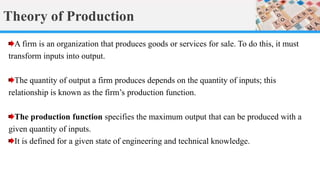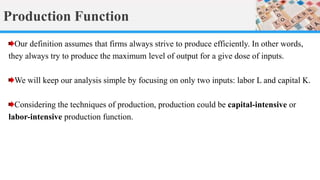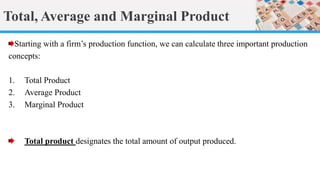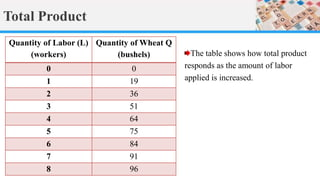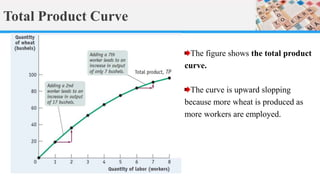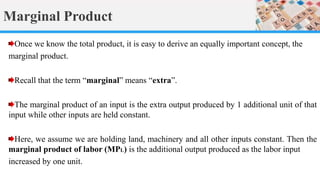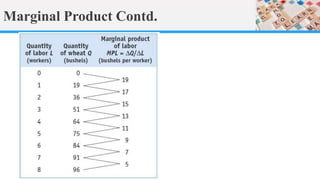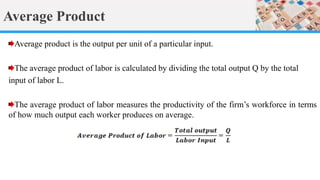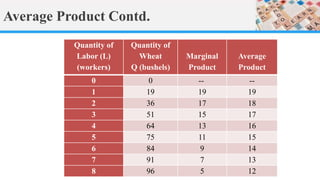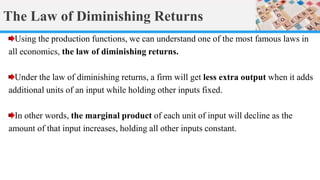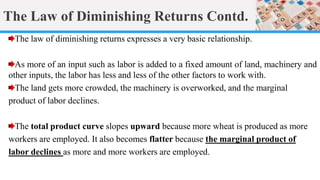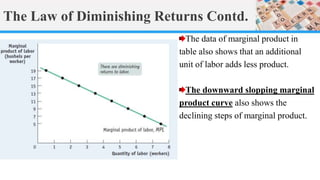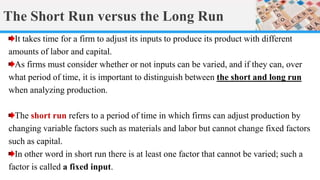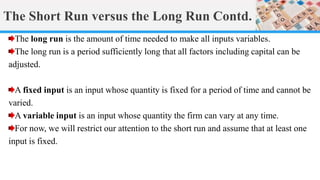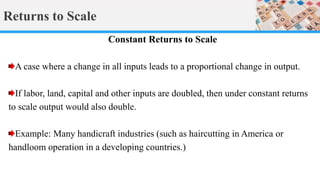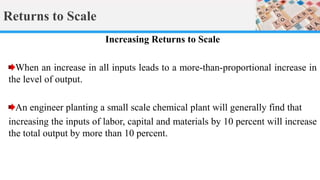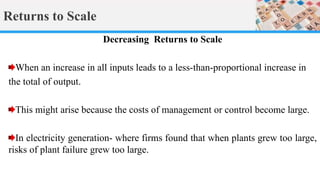1 de 18

### Production theory ECO 101

1. Production Theory Sumaiya Nabi Khan Principles of Microeconomics: ECO 101 Lecture 10
2. Theory of Production A firm is an organization that produces goods or services for sale. To do this, it must transform inputs into output. The quantity of output a firm produces depends on the quantity of inputs; this relationship is known as the firm’s production function. The production function specifies the maximum output that can be produced with a given quantity of inputs. It is defined for a given state of engineering and technical knowledge.
3. Production Function Our definition assumes that firms always strive to produce efficiently. In other words, they always try to produce the maximum level of output for a give dose of inputs. We will keep our analysis simple by focusing on only two inputs: labor L and capital K. Considering the techniques of production, production could be capital-intensive or labor-intensive production function.
4. Total, Average and Marginal Product Starting with a firm’s production function, we can calculate three important production concepts: 1. Total Product 2. Average Product 3. Marginal Product Total product designates the total amount of output produced.
5. Total Product The table shows how total product responds as the amount of labor applied is increased. Quantity of Labor (L) (workers) Quantity of Wheat Q (bushels) 0 0 1 19 2 36 3 51 4 64 5 75 6 84 7 91 8 96
6. Total Product Curve The figure shows the total product curve. The curve is upward slopping because more wheat is produced as more workers are employed.
7. Marginal Product Once we know the total product, it is easy to derive an equally important concept, the marginal product. Recall that the term “marginal” means “extra”. The marginal product of an input is the extra output produced by 1 additional unit of that input while other inputs are held constant. Here, we assume we are holding land, machinery and all other inputs constant. Then the marginal product of labor (MPL) is the additional output produced as the labor input increased by one unit.
8. Marginal Product Contd.
9. Average Product Average product is the output per unit of a particular input. The average product of labor is calculated by dividing the total output Q by the total input of labor L. The average product of labor measures the productivity of the firm’s workforce in terms of how much output each worker produces on average.
10. Average Product Contd. Quantity of Labor (L) (workers) Quantity of Wheat Q (bushels) Marginal Product Average Product 0 0 -- -- 1 19 19 19 2 36 17 18 3 51 15 17 4 64 13 16 5 75 11 15 6 84 9 14 7 91 7 13 8 96 5 12
11. The Law of Diminishing Returns Using the production functions, we can understand one of the most famous laws in all economics, the law of diminishing returns. Under the law of diminishing returns, a firm will get less extra output when it adds additional units of an input while holding other inputs fixed. In other words, the marginal product of each unit of input will decline as the amount of that input increases, holding all other inputs constant.
12. The Law of Diminishing Returns Contd. The law of diminishing returns expresses a very basic relationship. As more of an input such as labor is added to a fixed amount of land, machinery and other inputs, the labor has less and less of the other factors to work with. The land gets more crowded, the machinery is overworked, and the marginal product of labor declines. The total product curve slopes upward because more wheat is produced as more workers are employed. It also becomes flatter because the marginal product of labor declines as more and more workers are employed.
13. The Law of Diminishing Returns Contd. The data of marginal product in table also shows that an additional unit of labor adds less product. The downward slopping marginal product curve also shows the declining steps of marginal product.
14. The Short Run versus the Long Run It takes time for a firm to adjust its inputs to produce its product with different amounts of labor and capital. As firms must consider whether or not inputs can be varied, and if they can, over what period of time, it is important to distinguish between the short and long run when analyzing production. The short run refers to a period of time in which firms can adjust production by changing variable factors such as materials and labor but cannot change fixed factors such as capital. In other word in short run there is at least one factor that cannot be varied; such a factor is called a fixed input.
15. The Short Run versus the Long Run Contd. The long run is the amount of time needed to make all inputs variables. The long run is a period sufficiently long that all factors including capital can be adjusted. A fixed input is an input whose quantity is fixed for a period of time and cannot be varied. A variable input is an input whose quantity the firm can vary at any time. For now, we will restrict our attention to the short run and assume that at least one input is fixed.
16. Returns to Scale Constant Returns to Scale A case where a change in all inputs leads to a proportional change in output. If labor, land, capital and other inputs are doubled, then under constant returns to scale output would also double. Example: Many handicraft industries (such as haircutting in America or handloom operation in a developing countries.)
17. Returns to Scale Increasing Returns to Scale When an increase in all inputs leads to a more-than-proportional increase in the level of output. An engineer planting a small scale chemical plant will generally find that increasing the inputs of labor, capital and materials by 10 percent will increase the total output by more than 10 percent.
18. Returns to Scale Decreasing Returns to Scale When an increase in all inputs leads to a less-than-proportional increase in the total of output. This might arise because the costs of management or control become large. In electricity generation- where firms found that when plants grew too large, risks of plant failure grew too large.